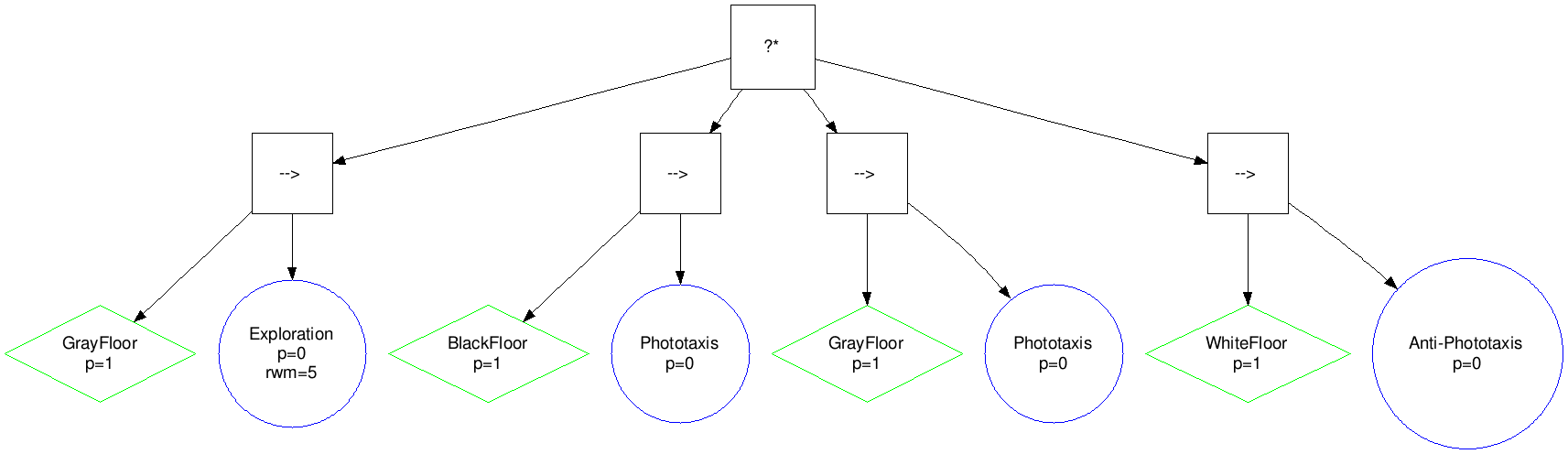# Automatic modular design of robot swarms using behavior trees as a control architecture

Antoine Ligot1, Jonas Kuckling1, Darko Bozhinoski1, Mauro Birattari1 (December 2018)

1IRIDIA, Université Libre de Bruxelles, Brussels, Belgium

 Table of Contents Study 1: performance in simulation and reality Study 2: performance version design budgets Study 3: Maple and some of its possible variants

In this page, you will find supplementary information about the three studies conducted. In the following, Sections 1, 2, and 3 are dedicated to Study 1, 2, and 3, respectively.

All the results obtained in the three studies are available for download here.

The source code used to generate the control software are available here: Maple, Chocolate, EvoStick

You can import them in R using read.csv("resultsAll.csv"). In these Sections you will find videos of robot experiments, images of the instances of control software generated by the design methods, and p-values tables. In Section 4, we illustrate the two instances of dummy control software. In Section 5, we illustrate behavior trees and finite-state machines that encodes an elaborate strategy for the Foraging mission.

In a p-values table, we report the p-values resulting of the assessment of Wilcoxon rank-sum tests in the left-hand part (all the cells (i, j) with j < i), and the corresponding relation between the two variables compared in th e right-hand part (that is, all the cells (i, j) with i < j). In some cases, the comparison between performances are not meaningfull: for example, comparing performance of two different design methods, one assessed in simulation, and the other one in reality. In these cases, we did not apply the Wilcoxon rank-sum test, and the corresponding cells contains the characters "NA". In the right-hand part, a cell can be empty, or have one of the following character: ">" or "<". If a cell (i, j) with i < j is empty, the corresponding p-value in cell (j, i) is greater than 0.05. If a cell (i, j) with i < j contains the character ">", the p-value in cell (j, i) is less than 0.05, and the variable of the i-th row is significantly greater than the variable of the j-th column. Similarly, if a cell (i, j) with i < j contains the character "<", the p-value in cell (j, i) is less than 0.05, and the variable of the i-th raw is significantly smaller than the variable of the j-th column. For example, in the p-values table of Study 1: Foraging, the cell (1, 2) contains ">", which means that the performance of Maple in simulation is significantly greater than the one of Maple in reality. Indeed, the corresponding p-value, reported in cell (2, 1), is equal to 4.66e-02, which is smaller than 0.05.

## 1. Study 1: performance in simulation and reality

### Foraging

The arena contains two source areas (black circles) and a nest (white area). A light is placed behind the nest to help the robots to navigate. In this idealized version of foraging, a robot is deemed to retrieve an object when it enters a source and then the nest. The goal of the swarm is to retrieve as many objects as possible.

Examples of experimental runs with robots, and examples of typical instances of control software, are displayed below. All videas and images of the control software are available for download: videos and control software.

#### AutoMoDe-Maple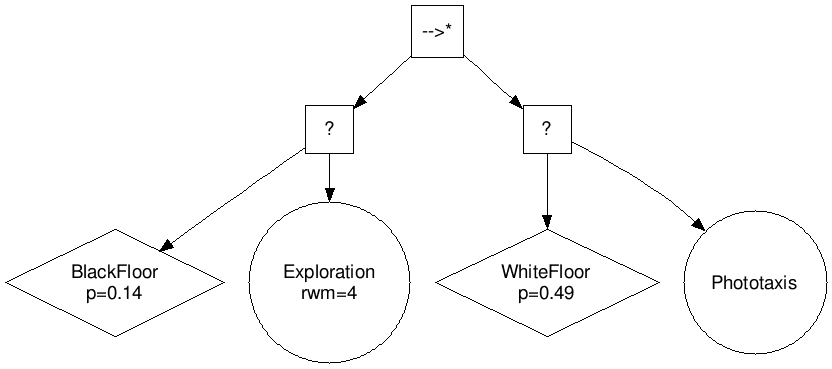#### AutoMoDe-Chocolate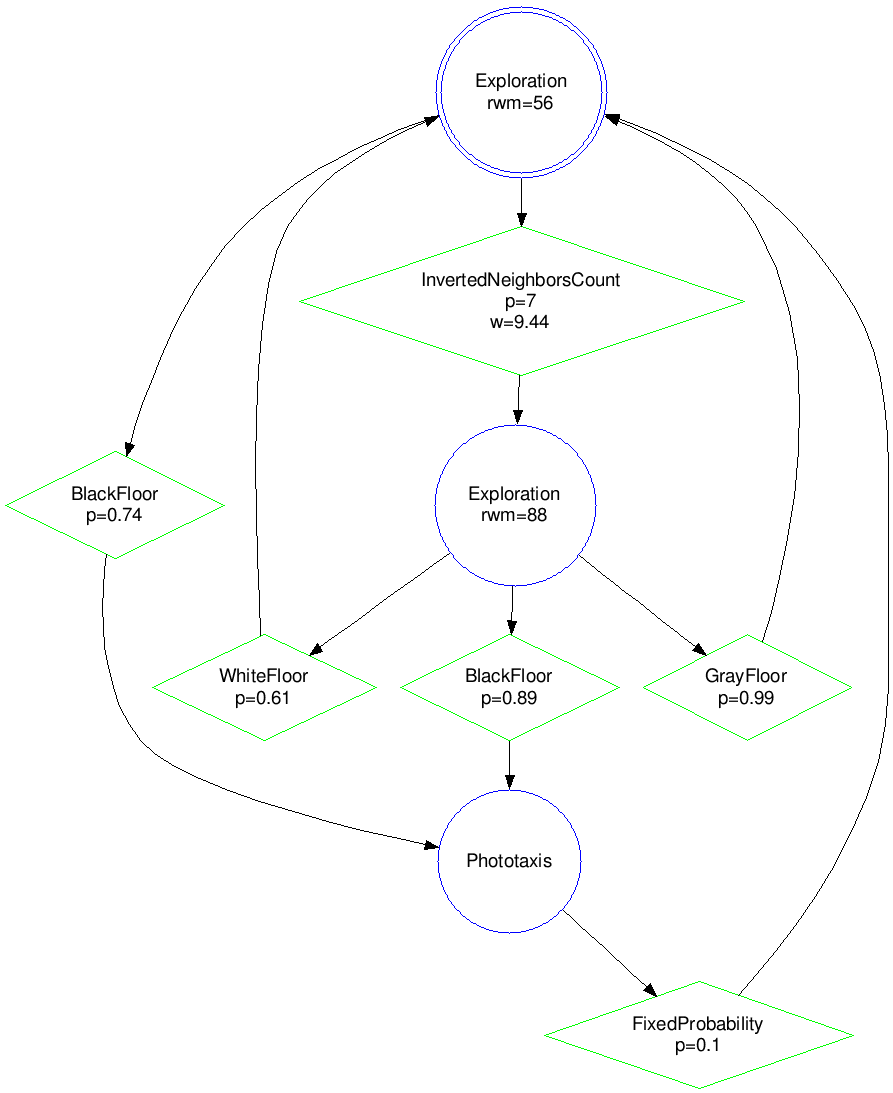#### P-values table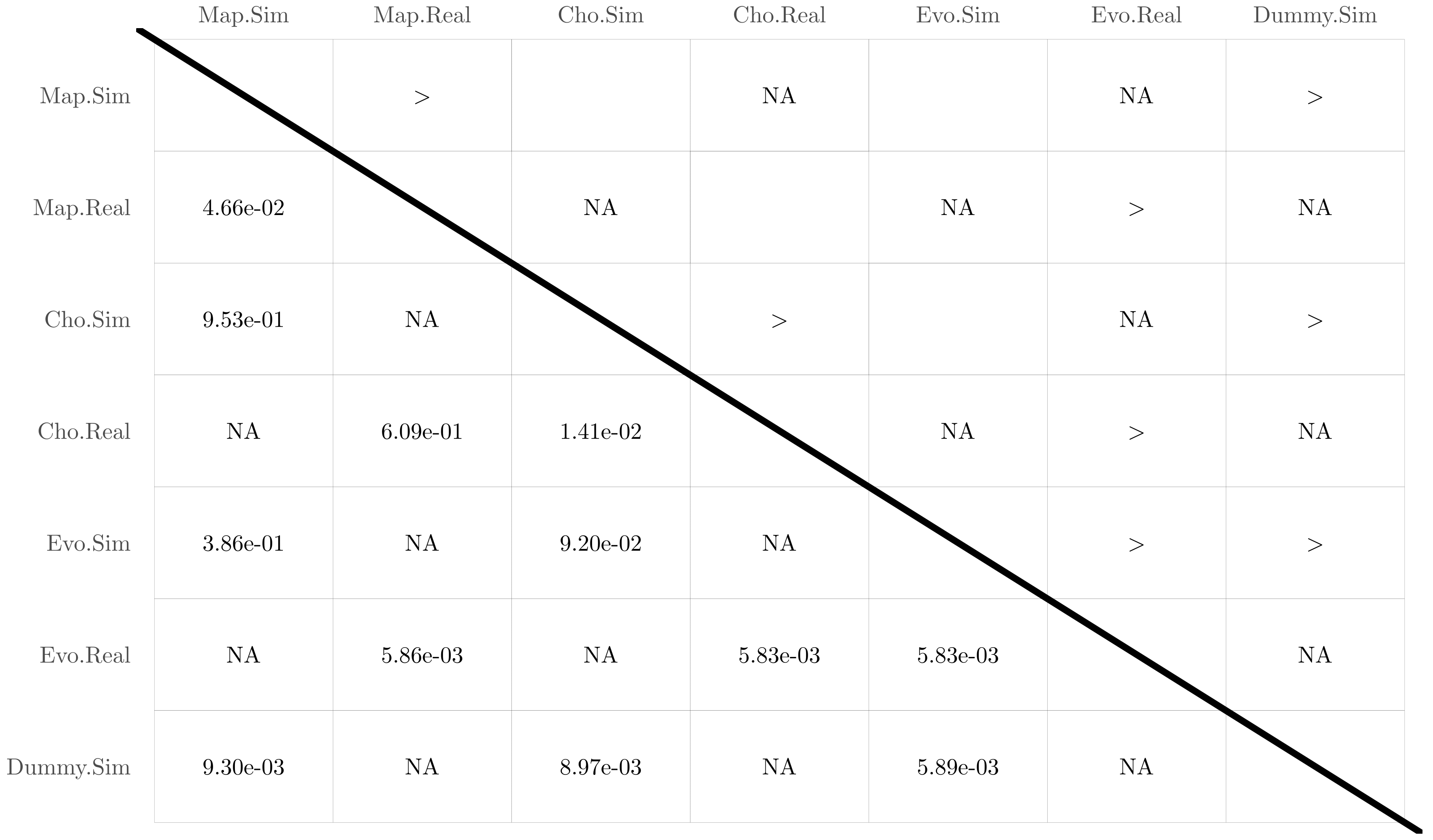### Aggregation

The swarm must select one of the two black areas and aggregate there. The objective function is computed at the end of the experimental run, and is maximized when all robots are either on the left or the right area.

Examples of experimental runs with robots, and examples of typical instances of control software, are displaye d below. All videas and images of the control software are available for download: videos and control software.

#### AutoMoDe-Maple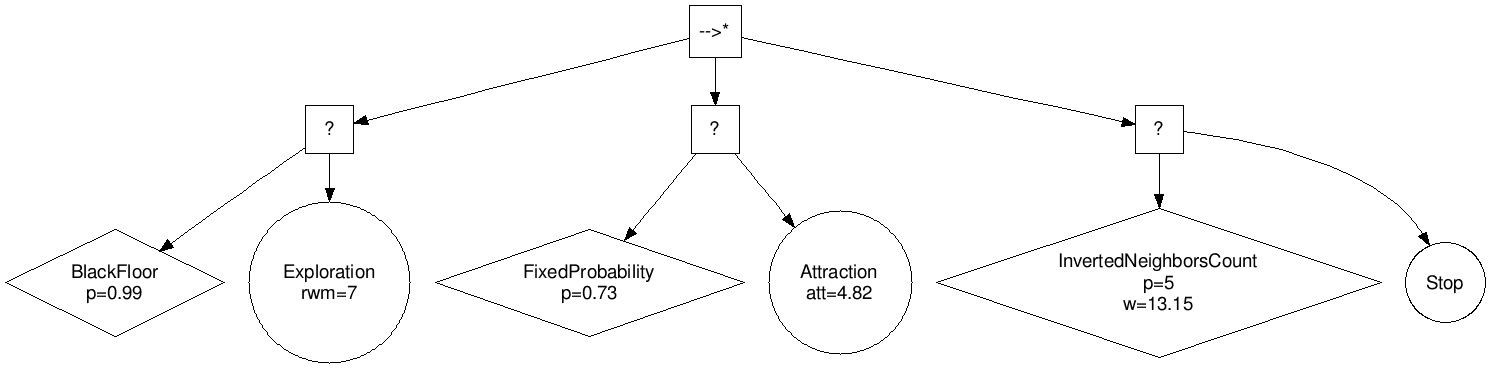#### AutoMoDe-Chocolate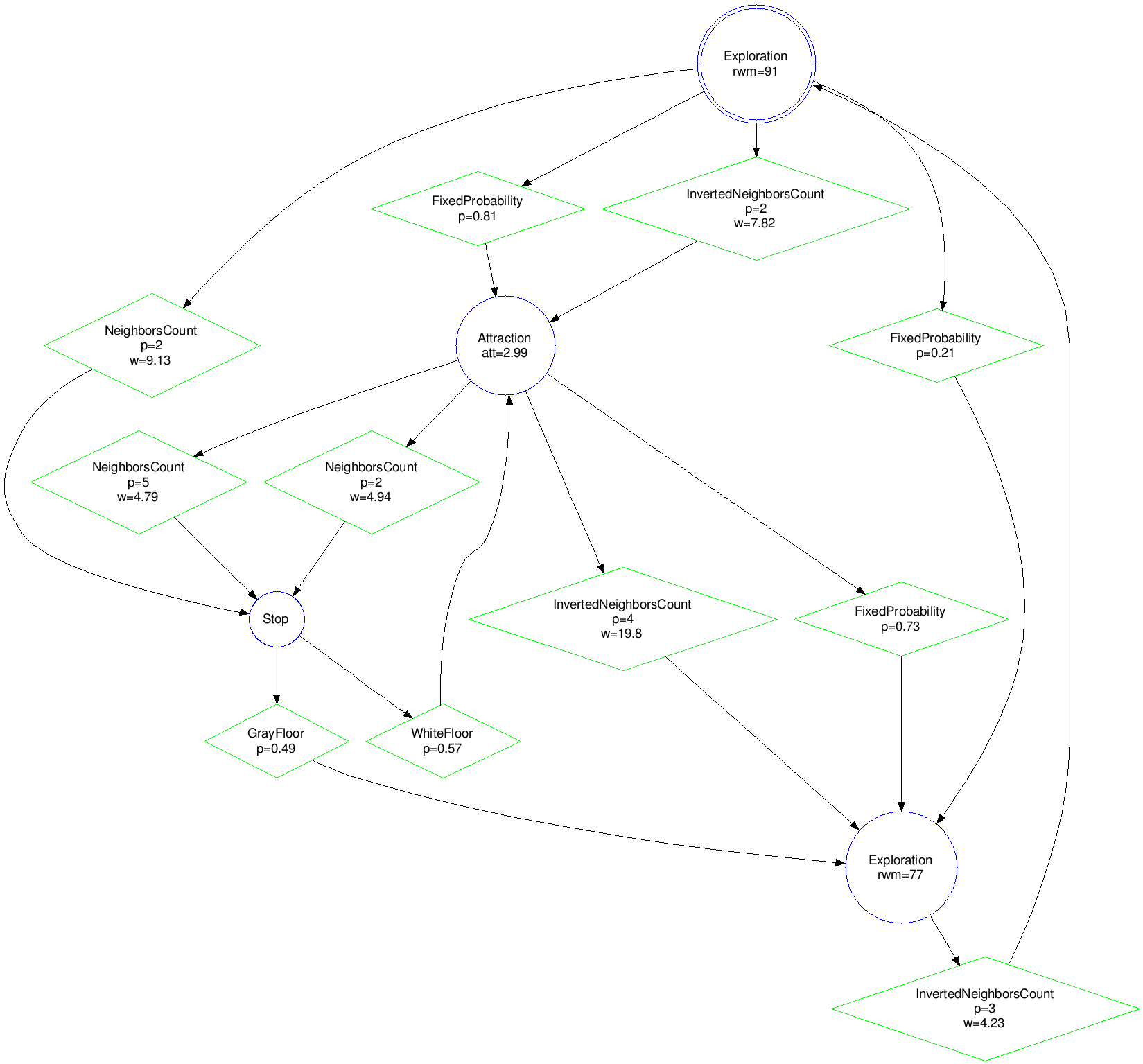#### P-values table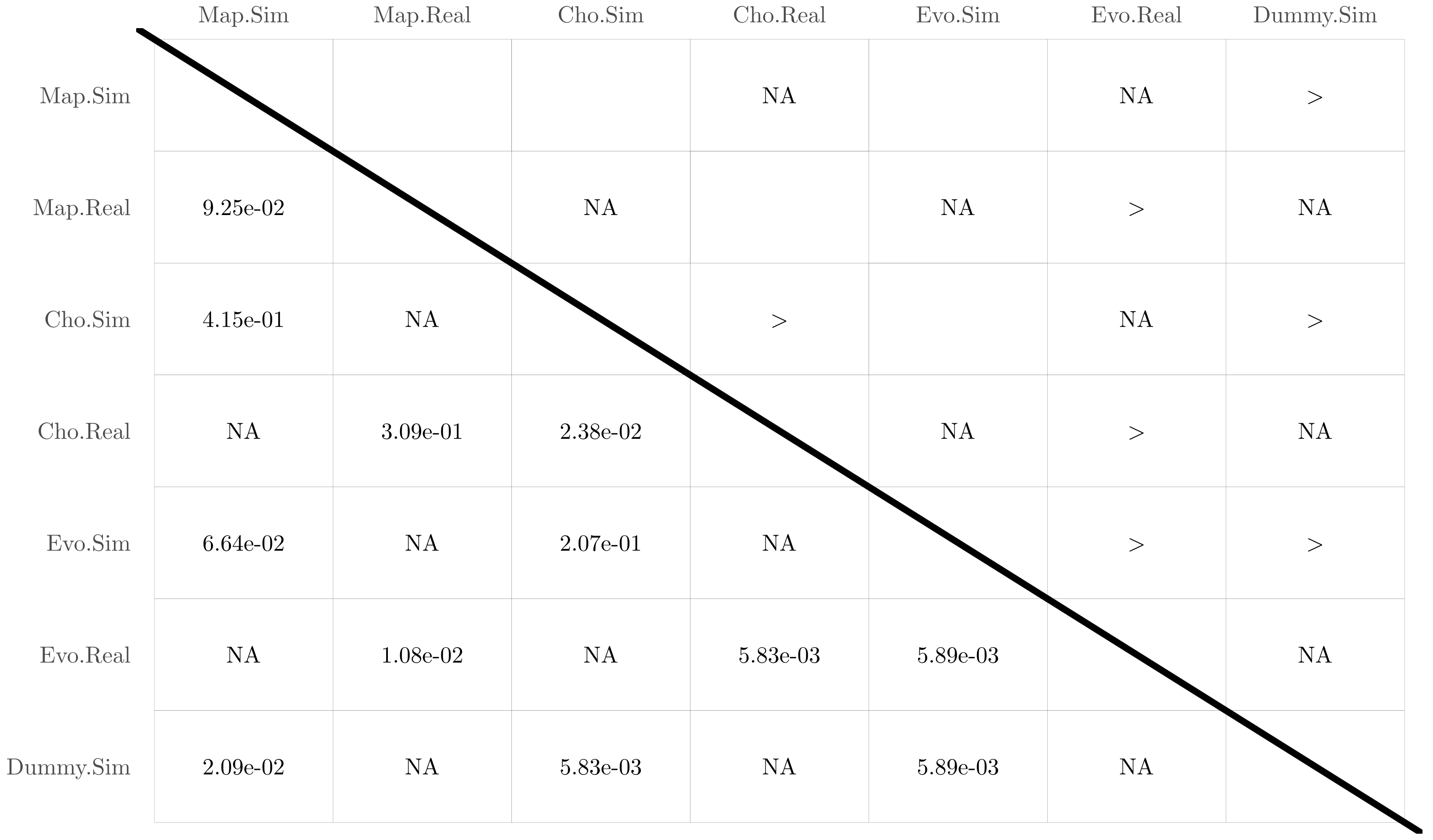## 2. Study 2: performance versus design budgets

In a p-values table, we report the p-values resulting of the assessment of Wilcoxon rank-sum tests in the left-hand part (all the cells (i, j) with j < i), and the corresponding relation between the two variables compared in the right-hand part (that is, all the cells (i, j) with i < j). In the right-hand part, a cell can be empty, or have one of the following character: ">" or "<". If a cell (i, j) with i < j is empty, the corresponding p-value in cell (j, i) is greater than 0.05. If a cell (i, j) with i < j contains the character ">", the p-value in cell (j, i) is less than 0.05, and the variable of the i-th row is significantly greater than the variable of the j-th column. Similarly, if a cell (i, j) with i < j contains the character "<", the p-value in cell (j, i) is less than 0.05, and the variable of the i-th raw is significantly smaller than the variable of the j-th column. For example, in the p-values table of Foraging, the cell (4, 5) contains "<", which means that Chocolate-1k is significantly worse thant Maple-5k. Indeed, the corresponding p-value, reported in cell (5, 4), is equal to 2.65e-03, which is smaller than 0.05.

#### P-values table Foraging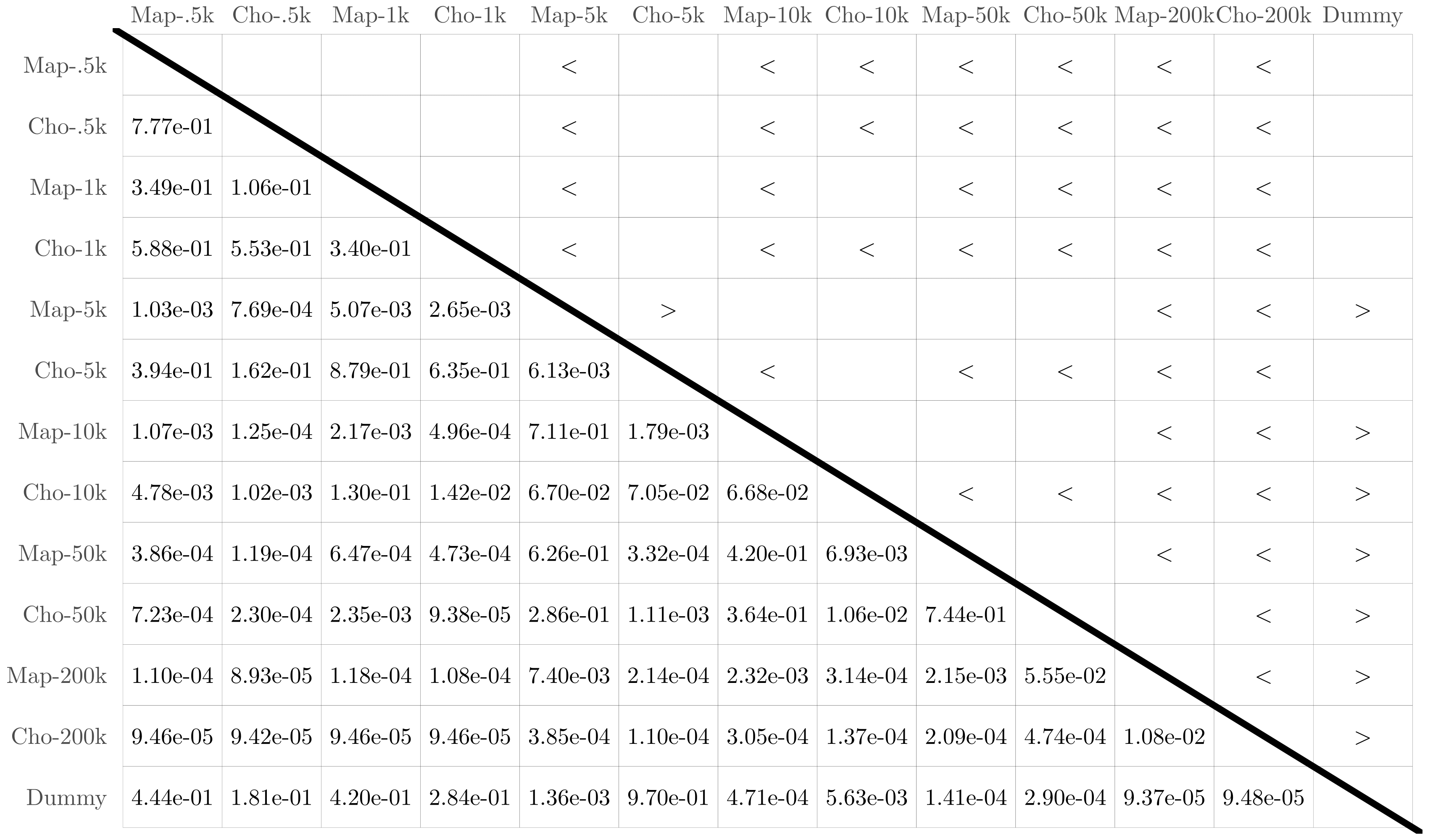#### P-values table Aggregation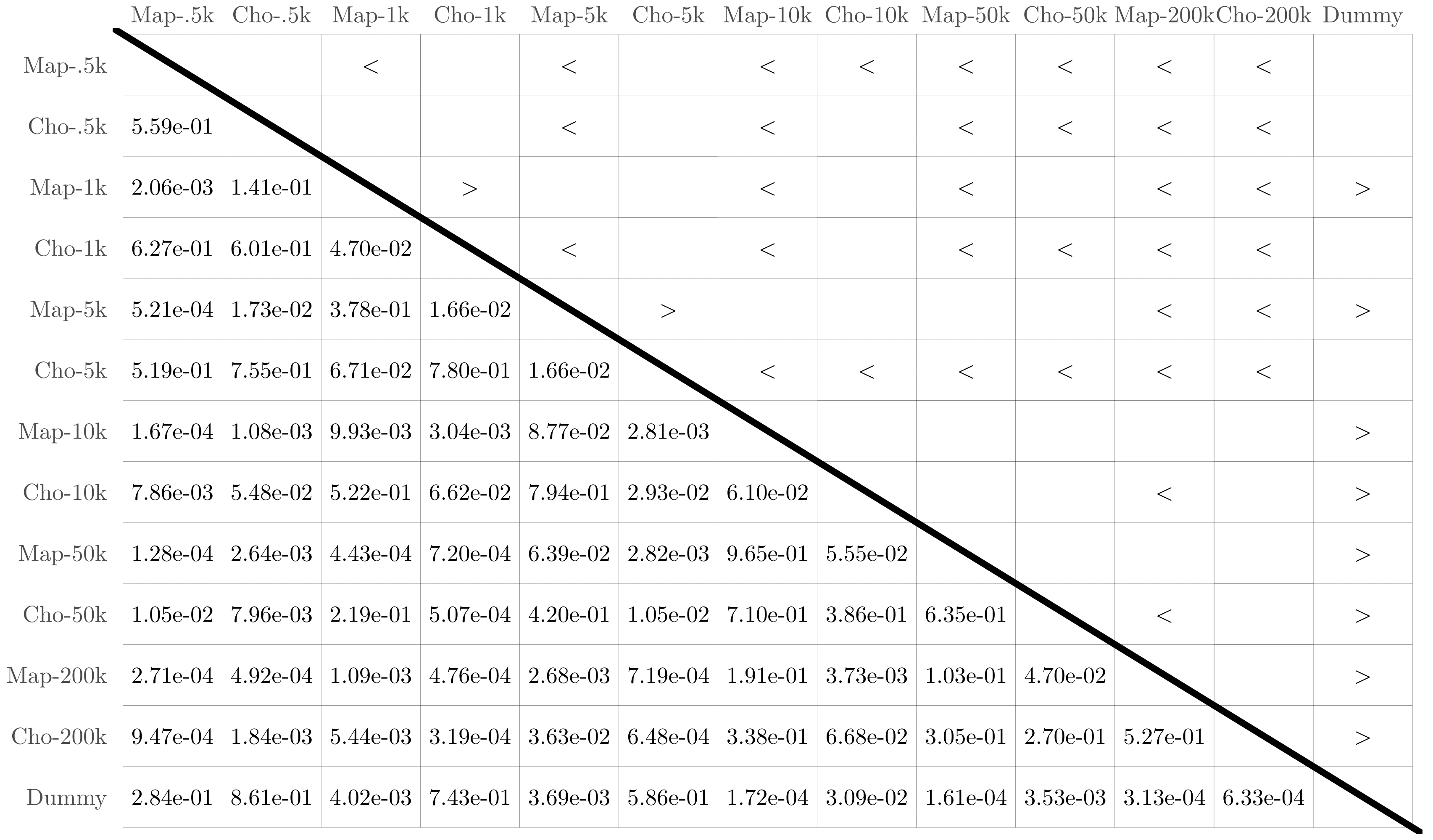## 3. Study 3: Maple and some of its possible variants#### Variant →*(→)#### Variant ?*(?)#### Variant →(?)#### Variant ?*(→)#### Variant →*(→|?)#### Variant ?*(→|?)

Here, we describe some variations of the class of behavior trees generated by Maple. The above figures illustrate behavior trees for which the leaf nodes are kept unchanged, but the inner node types may vary with respect to those of Maple. To refer to these variants, we use the following notation: ❬top❭ (❬inner❭) where ❬top❭ and ❬inner❭ are the types of the top-level node and the inner nodes, respectively. With this notation, the class of behavior trees produced by Maple can be formally indicated as →*(?)—the top-level node is a sequence* and the inner nodes are selector nodes. If the inner nodes of a variant can be chosen from 2 different types, we refer to it as htopi (❬inner1❭|❬inner2❭). For example, each inner node of the variant →*(→|?) can be either a sequence or a selector node. We consider the following variants:
→* (→): The top-level node is a sequence* node and the inner nodes are sequence nodes. This variant is not interesting as only action A1 can be executed, granted that condition C1 returns success. If condition C1 returns failure, no action is executed.
?*(?): The top-level node is a selector* node and the inner nodes are selector nodes. See Figure 6b. This variant is not interesting as only action A1 can be executed, granted that condition C1 returns failure. If condition C1 returns success, no action is executed.
→(?): The top-level node is a sequence node and the inner nodes are selector nodes. See Figure 6c. This variant is similar to →*(?) of Maple. However, because the top level node does not remember which subtree last returned running, the history of the past events is lost. In order for an action to be executed, all conditions situated to its left need to return success. Due to the absence of memory, we do not consider this variant promising|and neither any other memory-less one.
?*(→): The top-level node is a selector* and the inner nodes are sequence nodes. See Figure 6d. In this variant, the action node of a subtree is executed as long as the condition returns success, whereas it is executed until the condition returns success in Maple.
→* (→|?): The top-level node is a sequence* and the inner nodes can be sequence or selector nodes. See Figure 6e. This variant can produce meaningful behavior trees only if the inner nodes are selector nodes, which is exactly like in Maple's →*(?). Indeed, if the inner node of a given subtree is a sequence and the condition returns failure, then the execution of the behavior tree terminates. At the following iteration, the leftmost branch of the top-level node is ticked. Therefore, branches situated at the right of a sequence node are not likely to be ticked.
?*(→|?): The top-level node is a selector* and the inner nodes can be sequence or selector nodes. See Figure 6f. This variant can produce meaningful behavior trees only if the inner nodes are selector nodes, which is exactly like variant ?*(→). Indeed, if the inner node of a given subtree is a selector and the condition returns success, then the execution of the be havior tree terminates. At the following iteration, the leftmost branch of the top-level node is ticked. Therefore, branches situated at the right of a selector node are not likely to be ticked.#### Variant FL (free leaves)#### Variant ND (negation decorator)

In the following, we consider variants in which the inner nodes are kept unchanged, but the leaves are modied with respect to those of Maple. We illustrate some of these variants in the above figures.
FL (free leaves): Each leaf node is to be chosen between condition and action nodes. See Figure "Variant FL". Four pairs of leaf nodes are possible: condition–condition (see first branch), condition–action (which corresponds to the leaf pair imposed in Maple, see second branch), action–condition (see third branch), and action–action (see fourth branch). For each subtree, the optimization algorithm is free to chose any pair of leaf nodes. The variant can express disjunction of conditions: a branch following a condition–condition leaf pair is ticked if the first or the second condition is met. However, the variant introduces dead-end states: when an action on the left hand side of a leaf pair is ticked, the action is executed for the remaining of the simulation run.
CA|CC (condition–action or condition–condition): The right-hand side leaf node can be a condition or an action node. Two pairs of leaf nodes are thus possible: condition–action, condition–condition. With respect to FL, this variant can also express disjunction of conditions, but does not allow for dead-end states.
ND (negation decorator): A negation decorator node can be instantiated above a condition node. See Figure "Variant NL". The negation decorator returns failure (success) if the condition returns success (failure). With the set of conditions available, it is particularly interesting to place a negation decorator above a condition on the color of the ground perceived (that is black-, gray-, or white- floor). Indeed, placing a negation decorator node above a neighbor-count condition is equivalent to having an inverted-neighbor- count condition, and vice versa. Similarly, a negation decorator above a fixed-probability condition with p is equivalent to a fixed-probability with 1 - p. However, a negation decorator above a condition on a given color is equivalent to assessing the conditions for the two other colors simultaneously.
SP (success probability): This variant adopts Maple's →*(?), but each action node has a probability p to return success. The probability p is a real value in the range [0; 1] and is tuned by the optimization process. With this probability, we simulate the capability of the action nodes to assess if the low-level behaviors are successfully executed.

#### P-values table Foraging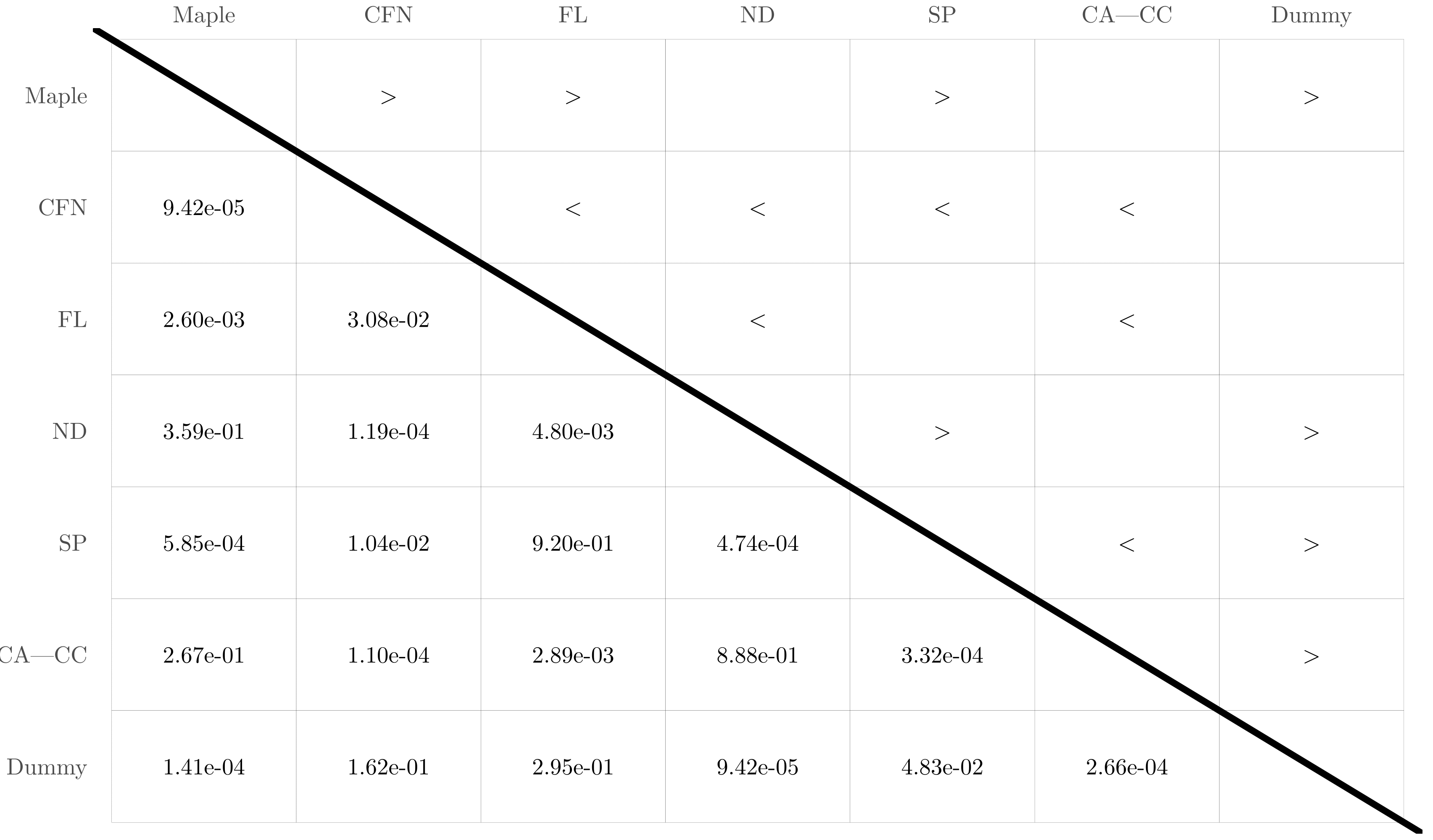#### P-values table Aggregation## 4. Dummy control software

Illustrations of the two instances of dummy control software. To fine-tune the parameters of the modules, we used Iterated F-race with a design budget of 1k simulation runs.

#### Foraging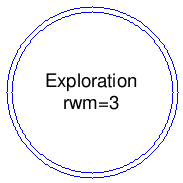#### Aggregation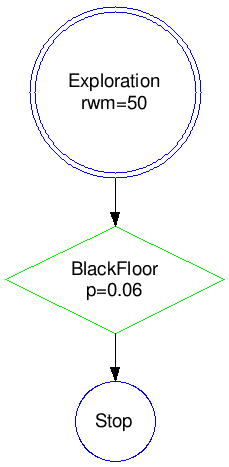## 5. Hand-made finite-state machines and behavior trees

Illustrations of behavior trees and finite-state machines that encodes the following individual behavior: the robot explores the gray area to find the food sources. Once a food source is found, the robot goes towards the light placed behind the nest using the phototaxis low-level behavior. If the robot enters the nest, it directly leaves it using the anti-phototaxis low-level behavior.

In the illustrations of behavior trees, the parameter p is the success probability (the probability that an action node return success). In Maple and all other variants with the exception of SP, the success probability is fixed to 0.

#### Finite-state machine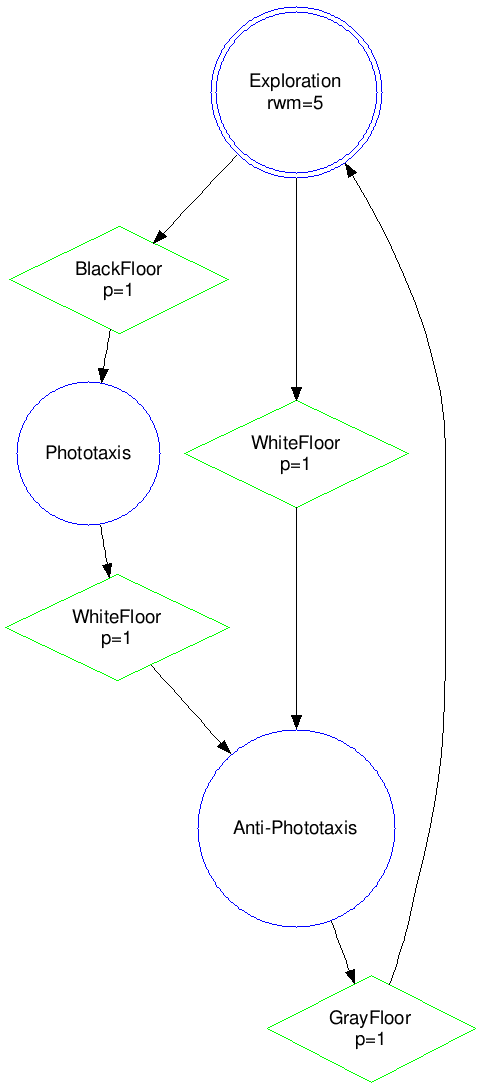#### Behavior tree variant ND (negation decorator)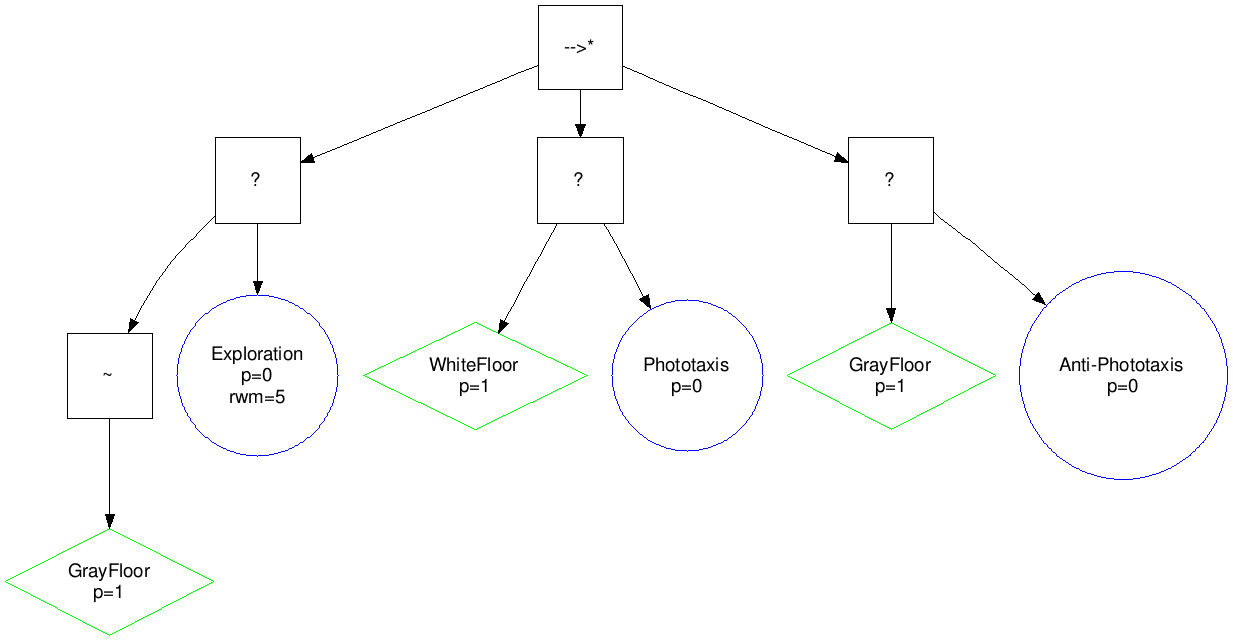#### Behavior tree variant CFN (control-flow node)